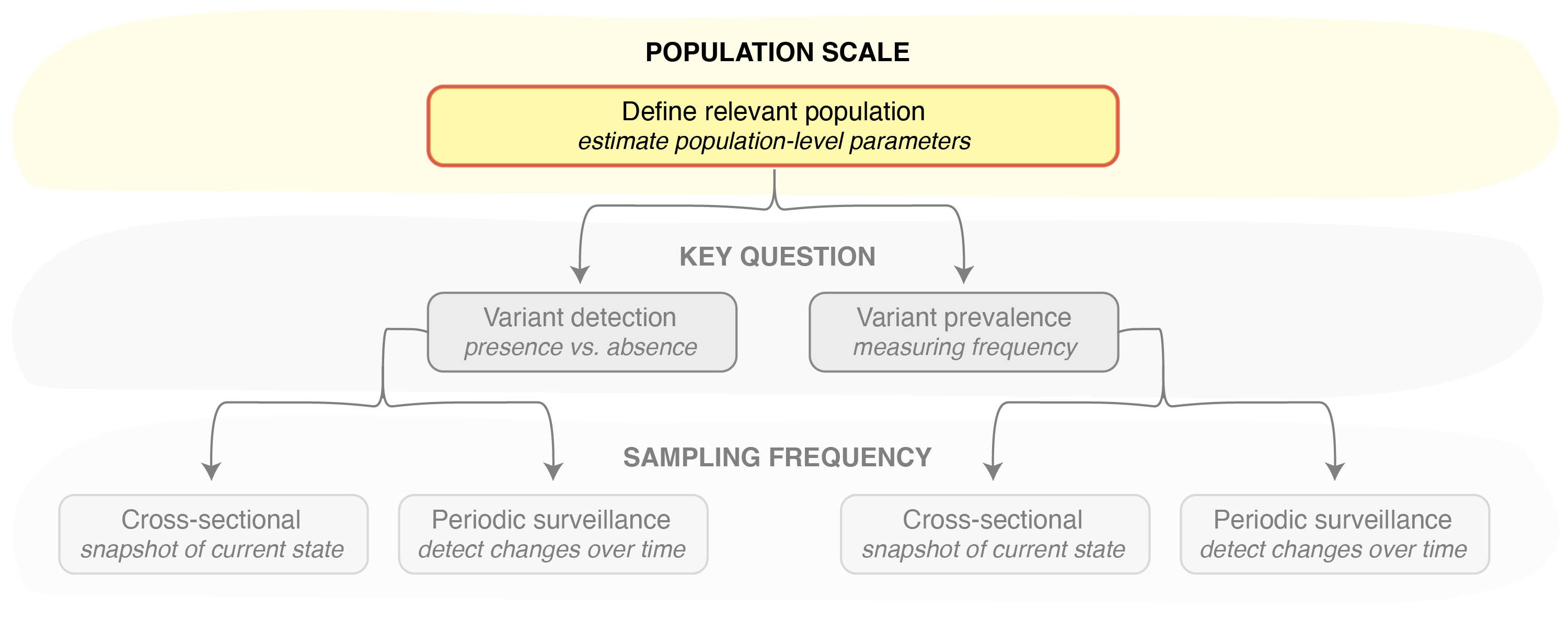# Estimating bias in observed variant prevalence

This vignette provides an overview of functions that are useful for understanding bias in the detection of pathogen variants and estimation of their prevalence in a population. These functions are important because defining the population and estimating the parameters that may bias variant surveillance is often the first step in estimating the sample size needed to answer the questions at hand (Figure 1).Figure 1. Defining the population of interest and approximating population- and variant-specific parameters is the first step in designing any pathogen variant surveillance program.

Central to these functions is the assumption that biological differences in pathogen variants may affect how infections are tested and characterized. For example, a variant that causes more severe clinical outcomes may result in a higher testing rate, since more individuals get sick enough to present at a healthcare facility. This higher testing rate may mean that a higher proportion of the samples selected for molecular characterization (e.g., sequencing) belong to this variant than the true underlying frequency of this variant in the population.

The combination of the factors that may affect the detection and characterization of a particular variant can be summarized in a parameter termed the coefficient of detection, which in turn can be estimated from the following parameters. This is not a comprehensive list of parameters, but rather the parameters currently implemented in the phylosamp package, since they are likely to have a significant effect on variant detection and prevalence estimation:

Param Variable Name Description
$$\phi_{V_1}$$ phi_v1 the probability that a tested infection caused by variant 1 results in a positive test (sensitivity)
$$\phi_{V_2}$$ phi_v2 the probability that a tested infection caused by variant 2 results in a positive test (sensitivity)
$$\gamma_{V_1}$$ gamma_v1 the probability that a detected infection caused by variant 1 meets some quality threshold
$$\gamma_{V_2}$$ gamma_v2 the probability that a detected infection caused by variant 2 meets some quality threshold
$$\psi_{V_1}$$ psi_v1 the probability that an infection caused by variant 1 is asymptomatic
$$\psi_{V_2}$$ psi_v2 the probability that an infection caused by variant 2 is asymptomatic
$$\tau_{a}$$ tau_a the probability of testing an asymptomatic infection (any variant)
$$\tau_{s}$$ tau_s the probability of testing a symptomatic infection (any variant)

While it may be difficult to estimate all of the parameters needed to estimate this coefficient (for examples on how to approximate these parameters in real-world scenarios, see Variant surveillance example vignette), we find that only the ratio in the coefficients of detection for two variants (more commonly, the variant of interest and the values for the rest of the pathogen population) is necessary for understanding the biases in a sample. Therefore, it may not be necessary to estimate the true value of each parameter, provided we can estimate the ratio between their values for the two variants in question. We can use the vartrack_cod_ratio() function to estimate the coefficient of detection ratio, only providing parameters which are expected to differ between variants. The ratio between any variants not provided is assumed to be equal to one.

library(phylosamp)
vartrack_cod_ratio(phi_v1=0.975, phi_v2=0.95, gamma_v1=0.8, gamma_v2=0.6)
##  1.368421

In this case, we assume that the testing sensitivity is higher for variant 1 ($$\phi_{V_1}=0.975$$) than it is for the rest of the pathogen population ($$\phi_{V_2}=0.95$$). Similarly, we assume that samples containing variant 1 have a higher chance of characterization success (by sequencing or another method; $$\gamma_{V_1}=0.8$$) than other samples ($$\gamma_{V_2}=0.6$$).

We also assume that the asymptomatic rate is the same between variants, and thus this parameter does not need to be provided. When the asymptomatic rate is the same between variants, the testing probability (which could differ for symptomatic and asymptomatic infections, but is assumed to be the same between variants, since the variant is not usually known at the time of testing) no longer affects the coefficient of detection ratio, and thus also does not need to be provided.

With the coefficient of detection ratio in hand, we can now use other package functions to estimate how this ratio will affect our observed variant frequency. For example, we can use the varfreq_obs_freq() function to estimate how changing parameters such as the testing sensitivity, testing frequency, asymptomatic rate, etc. will affect the variant frequency we expect to see in our pool of tested samples, given some underlying true variant prevalence:

varfreq_obs_freq(p_v1=0.2, c_ratio=1.368)
##  0.2548435

Given the coefficient of detection ratio calculated above ($$1.368$$) we can see that we would observe the variant of interest at approximately 25% prevalence in any well-mixed sample, even though the true underlying prevalence is only 20%. This enrichment may reduce the sample size needed to detect the variant when present at low frequencies, but may make it difficult to estimate changes in the variant frequency with precision.

We can use a separate function to estimate the multiplicative bias in the observed variant prevalence estimated above—in other words, the observed variant prevalence divided by the true variance prevalence:

varfreq_expected_mbias(p_v1=0.2, c_ratio=1.367)
##  1.273523

As expected, this value is the result from the varfreq_obs_freq() function divided by the true underlying prevalence. This value tells use that we are overestimating the variant of interest frequency by roughly 25%.

##### Sample size calculations

To learn more about the package functions that can be used to calculate the sample size needed to detect or monitor a variant given different sampling scenarios, see the Estimating the sample size needed for variant monitoring cross-sectional and periodic sampling vignettes. To learn more about the package functions that can be used to estimate the probability of variant detection, see the Estimating the probability of detecting a variant cross-sectional and periodic sampling vignettes.